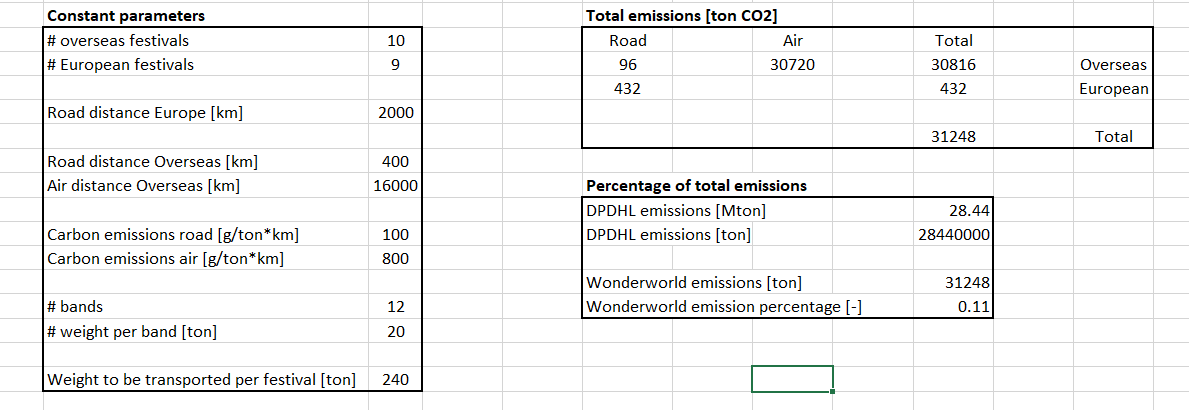# Can someone help me to understand the calculations?

DHL Consulting Case: Wonderworld Music Festival
New answer on Nov 13, 2020
2.2 k Views

I went through this case and found out that the calculations were strange.

Exhibit 3: DPDHL Carbon Footprint 2017 - the calculations are abit off.

For example, based on my calculation, the total emission should be 2.1 + 9.7 + 14.7 + 2.3 = 28.8 instead of 28.44.

Exhibit 4: Calculation Quantitative Analysis - I cant follow the logic; I think the way the author do the computation on excel was poor. Furthermore, I still managed to follow the flow, however, I couldnt get the same results.

1. How to calculate Total Emission for Road and Air?

2. How to get the Total Emission for Overseas Festivals? Should it be a basic summation of Road and Air?

Can someone enlighten me? :)

• Date ascending
• Date descending

I agree that the computations in this case are completely off, however I also cannot agree with your computation.

I found the following:
- 1.5 + 0.6 = 2.1
- 5 + 4.7 = 9.7
- 14.5 + 0.1 = 14.6
- 1.5 + 0.8 = 2.3

- 2.1 + 9.7 + 14.6 + 2.3 = 28.7

I translated the other quantitative part of this case into an excel file which I cannot upload here unfortunately, so you will have to deal with this screenshot.

The numbers below should be the correct answers to the following two questions:
- What is the total amount of CO2 emissions for the festival: 31248 ton
- What percentage of the total carbon footprint of DPDHL is taken up by the festival: 0.11% (note that this percentage is calculated based on a total emission of 28.44 Mton instead of the correct 28.7 Mton. However, the difference is so small that due to rounding the percentage would still be 0.11%)

If we look at exhibit 4 we can see some strange things happening. For some reason the total emissions for the transportation over the road for the European festivals seem to magically change from 756 to 436 ton. This is simply impossible.

I hope this anwer prevents any further victims of this vague case.

Good luck practising!